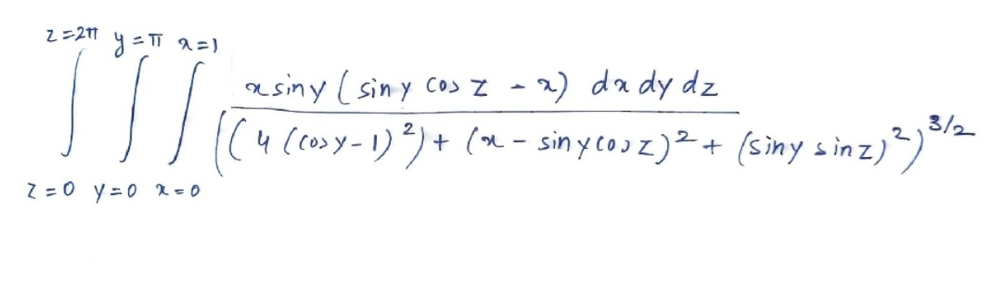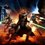# That really complicated integral lol

  1 2 3 4 5 6 7 8 9 10 11 12 13 14 15 16 17 18 19 20 21 22 23 24 25 26 27 28 29 30 31 32 33 34 import math dx = 10**-6 dy = 10**-6 dz = 10**-6 x = 0.1 y = 0.1 z = 0.1 def tripleVariableFunction(x,y,z): numerator = x*math.sin(y)*(math.sin(y)*math.cos(z)-x) denominator = (4*(math.cos(y)-1)**2 + (x-math.sin(y)*math.cos(z))**2 + (math.sin(y)*math.sin(z))**2)**(1.5) return numerator/denominator xIntegral = 0 yIntegral = 0 zIntegral = 0 while z <= 6.28: while y <= 3.14: while x <= 1: xIntegral += tripleVariableFunction(x,y,z)*dx x += dx yIntegral += xIntegral*dy y += dy zIntegral += yIntegral*dz z += dz print(zIntegral)So, I think the integral is divergent because at $x = 0, y = 0, z = 0$ the function value goes to infinity. The integral value, of course, skyrockets. So, I have tried running the integral as close to zero as possible. It is very clear how the integral value increases by crazy amounts when $x,y,z \rightarrow 0$, whereas when the values are 0.1 the integral is much smaller.Note by Krishna Karthik
2 weeks, 1 day ago

This discussion board is a place to discuss our Daily Challenges and the math and science related to those challenges. Explanations are more than just a solution — they should explain the steps and thinking strategies that you used to obtain the solution. Comments should further the discussion of math and science.

When posting on Brilliant:

• Use the emojis to react to an explanation, whether you're congratulating a job well done , or just really confused .
• Ask specific questions about the challenge or the steps in somebody's explanation. Well-posed questions can add a lot to the discussion, but posting "I don't understand!" doesn't help anyone.
• Try to contribute something new to the discussion, whether it is an extension, generalization or other idea related to the challenge.

MarkdownAppears as
*italics* or _italics_ italics
**bold** or __bold__ bold
- bulleted- list
• bulleted
• list
1. numbered2. list
1. numbered
2. list
Note: you must add a full line of space before and after lists for them to show up correctly
paragraph 1paragraph 2

paragraph 1

paragraph 2

[example link](https://brilliant.org)example link
> This is a quote
This is a quote
    # I indented these lines
# 4 spaces, and now they show
# up as a code block.

print "hello world"
# I indented these lines
# 4 spaces, and now they show
# up as a code block.

print "hello world"
MathAppears as
Remember to wrap math in $$ ... $$ or $ ... $ to ensure proper formatting.
2 \times 3 $2 \times 3$
2^{34} $2^{34}$
a_{i-1} $a_{i-1}$
\frac{2}{3} $\frac{2}{3}$
\sqrt{2} $\sqrt{2}$
\sum_{i=1}^3 $\sum_{i=1}^3$
\sin \theta $\sin \theta$
\boxed{123} $\boxed{123}$

Sort by:

@Krishna Karthik i have made some changes in the code
and now the answer is $-15.15$

  1 2 3 4 5 6 7 8 9 10 11 12 13 14 15 16 17 18 19 20 21 22 23 24 25 26 27 28 29 30 31 32 33 34 35 36 37 38 39 import math dx = 10**-6 dy = 10**-6 dz = 10**-6 x = 0.01 y = 0.01 z = 0.01 def tripleVariableFunction(x,y,z): numerator = x*math.sin(y)*(math.sin(y)*math.cos(z)-x) denominator = (4*(math.cos(y)-1)**2 + (x-math.sin(y)*math.cos(z))**2 + (math.sin(y)*math.sin(z))**2)**(1.5) return numerator/denominator xIntegral = 0 yIntegral = 0 zIntegral = 0 while z <= 6.28: while y <= 3.14: while x <= 1: xIntegral += tripleVariableFunction(x,y,z)*dx x += dx yIntegral += xIntegral*dy y += dy zIntegral += yIntegral*dz z += dz print(zIntegral) # ========================== RESTART: E:\test.py (2).txt ========================= # -15.551517796734224 

- 2 weeks, 1 day ago

Ok, so you have adjusted accuracy and brought the values of x,y and z closer to 0. But that doesn't change the fact that $f(x,y,z)$ is undefined at $x,y,z$ = 0

- 2 weeks, 1 day ago

@Krishna Karthik but if we want a perfect answer than ,then we have to do that as much as we want

by the way how to write that in python in brilliant ,i just forgot

- 2 weeks, 1 day ago

That's ok, just type three characters that look like this: 

Above and below your code, on new lines. At the top after the three character thing, on the same line, type "python".

- 2 weeks, 1 day ago

I have tried bringing x,y, and z closer to 0, but the value of the integral only gets greater (by large amounts). I think this proves its divergence.

- 2 weeks, 1 day ago

Bro I like your new profile pic and description. It screams: "Badass; rich guy"

- 15 hours ago

Thanks,by the way @Steven Chase seems me a badass guy.

- 15 hours ago

@Krishna Karthik I have posted the problem here
And this problem need this hard integral , which we were solving.

- 2 weeks, 1 day ago

But how? The integral is divergent. Did you actually manage to solve the integral?

- 2 weeks ago

@Krishna Karthik i was getting 0.4 many times, therefore I considered it as a right answer.

- 2 weeks ago

But the answer that was on the problem was 0.201

- 2 weeks ago

@Krishna Karthik after that integral my calculation says that we have to divide by 2

- 2 weeks ago

Ahh ok. Fair enough :)

- 2 weeks ago

Let's see what Steven Chase says about it :)

- 2 weeks ago

Sounds like a really cool question!!

- 2 weeks ago

@Krishna Karthik I think the answer should be positive.

- 2 weeks, 1 day ago

I'll check.

- 2 weeks, 1 day ago

There is one small thing: the function skyrockets to infinity when x = 0, y = 0, and z = 0

Personally, I think the integral is divergent.

- 2 weeks, 1 day ago

@Krishna Karthik i think when i will post this problem , it will be the hardest problem of whole electricty and magnetsim in brilliant.

- 2 weeks, 1 day ago

Lmao🤣

Good one bud

- 2 weeks, 1 day ago

What's hard about triple integrals is that since they are in 4 dimensions, you can't visualise them.

But I think evidence shows that the integral is divergent, much like $\displaystyle \int_{0}^{1} \frac{1}{x} dx$ is.

- 2 weeks, 1 day ago

@Krishna Karthik bro the answer is coming in 1 second ,how it is possible ,it is very hard integral??

- 2 weeks, 1 day ago

I was wrong about the computer's capability lmao. The number of iterations are manageable. Previously I thought it was too big, but that was just a code error.

- 2 weeks, 1 day ago

@Krishna Karthik i am surprising by new answer everytime

  1 2 3 4 5 6 7 8 9 10 11 12 13 14 15 16 17 18 19 20 21 22 23 24 25 26 27 28 29 30 31 32 33 34 35 36 37 38 import math dx = 10**-6 dy = 10**-6 dz = 10**-6 x = 0.0001 y = 0.0001 z = 0.0001 def tripleVariableFunction(x,y,z): numerator = x*math.sin(y)*(math.sin(y)*math.cos(z)-x) denominator = (4*(math.cos(y)-1)**2 + (x-math.sin(y)*math.cos(z))**2 + (math.sin(y)*math.sin(z))**2)**(1.5) return numerator/denominator xIntegral = 0 yIntegral = 0 zIntegral = 0 while z <= 6.28: while y <= 3.14: while x <= 1: xIntegral += tripleVariableFunction(x,y,z)*dx x += dx yIntegral += xIntegral*dy y += dy zIntegral += yIntegral*dz z += dz print(zIntegral) ====================== RESTART: E:\test.py (2).txt ====================== -0.39914323545949787 >>> `

- 2 weeks, 1 day ago

Ok, got it. Now I'm getting something around the 0.4 ballpark.

- 2 weeks, 1 day ago

@Krishna Karthik what do you mean by ballpark?

- 2 weeks, 1 day ago

Around the 0.4 mark. Around there.

- 2 weeks, 1 day ago

@Krishna Karthik bro so which answer is correct??

- 2 weeks, 1 day ago

Let me do some more testing.

- 2 weeks, 1 day ago

What??? I lowered the x y and z values toward zero more, and now I'm getting 47. ???

- 2 weeks, 1 day ago

@Krishna Karthik ha ha ha ha i laughed so hard by reading the above comment.

- 2 weeks, 1 day ago

Yeah; there's no doubt about it; the integral you gave me is divergent. You can see it clearly as the integral value skyrockets as x, y and z approach zero. And you have a division by zero error the compiler gives when x y and z = 0.

- 2 weeks, 1 day ago

@Krishna Karthik but why the integral is divergent ? the problem which i am making should definitey have some answer .

- 2 weeks, 1 day ago

Try to take a look at the integral again. Maybe there's some other answer, or something.

- 2 weeks, 1 day ago

Have you got the answer for the problem? How have you got the answer if the integral is incorrect?

- 2 weeks ago

Anyway, gtg. See ya.

- 2 weeks, 1 day ago

@Krishna Karthik what this above comment mean ??

- 2 weeks, 1 day ago

Gtg stands for "got to go"

- 2 weeks, 1 day ago

@Krishna Karthik so what about the integral. Did Steven sir helped you??

- 2 weeks ago

Not yet; I haven't heard from him

- 2 weeks ago

@Krishna Karthik let me the run the code $1000$ time by different values .
and then we will take the average of all those $1000$ values.ha ha ha

- 2 weeks, 1 day ago

Lol

- 2 weeks, 1 day ago

Bruh

- 2 weeks, 1 day ago

Ok, I might just ask Steven Chase or someone to confirm what's going on.

- 2 weeks, 1 day ago

So the integral you wished for me to calculate was wrong? That makes sense. I just saw the numerical solution by Steven Chase. It was quite brilliant.

- 2 weeks ago

@Krishna Karthik if we calculate my integral very accurately so may be we will get correct answer?

- 2 weeks ago